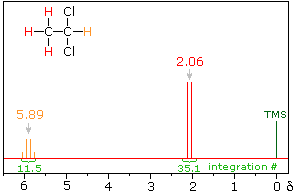# How can I draw NMR splitting diagrams?

Dec 17, 2014

The general rule for $\text{^1"H}$ NMR spectra is: n neighbouring protons with the same coupling constant J will split a signal into n+1 lines.

We draw a "splitting tree" for each proton or group of protons.No splitting. Draw a single line.

Draw 2 lines (a doublet) separated by ${J}_{a x}$.

From each line of the doublet, draw two more lines separated by ${J}_{a x}$. Stack the overlapping lines. This gives a triplet with area ratios 1:2:1.

From each line of the triplet, draw two more lines separated by ${J}_{a x}$. Stack the overlapping lines. This gives a quartet with area ratios 1:3:3:1.

Repeat the process as many times as needed.

The relative intensity ratios are given by Pascal's triangle.Here are some splitting patterns that you should be able to draw quickly.

AX doublet-doublet: -CH-CH-

AX₂ doublet-triplet: -CH-CH₂-AX₃ doublet-quartet: CH₃CH-AX₆ doublet-septet: (CH₃)₂CH- (an isopropyl group!)Note: A septet often looks like a quintet with the wrong intensities, because the two outermost lines are so weak.

A₂X₃ triplet quartet: CH₃CH₂- (an ethyl group!)Here's a video that gives an introduction to NMR splitting patterns.